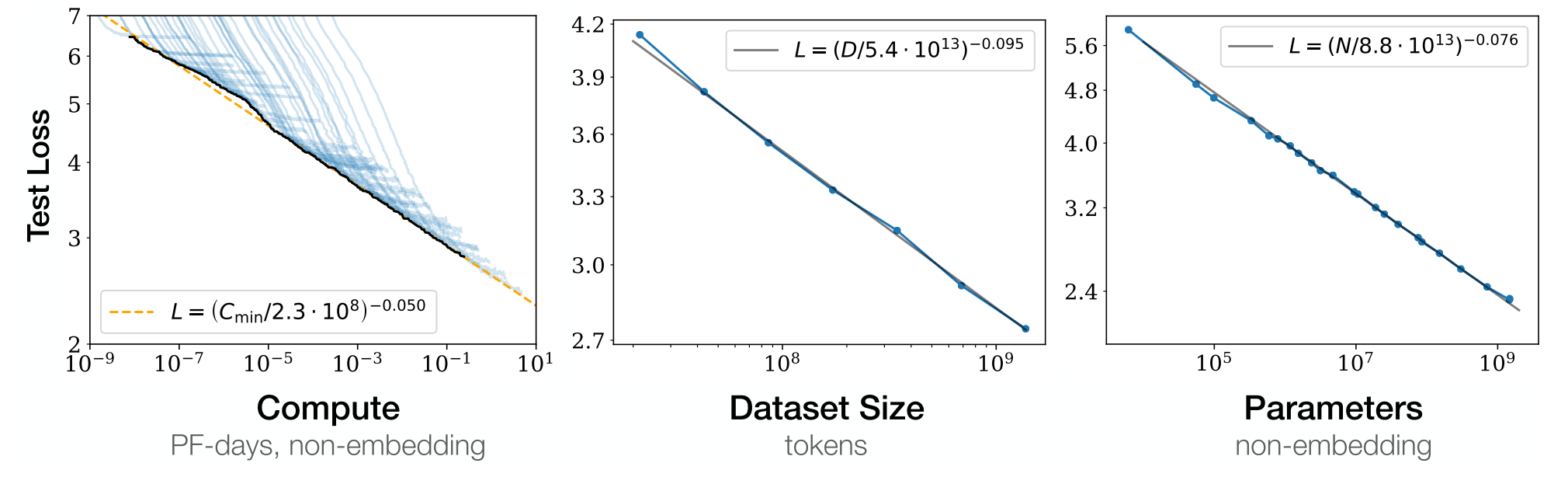# Scaling Laws for Neural Language Models

Kaplan et al., 2020Source: Kaplan et al., 2020

## Summary

• Study empirical scaling laws for language model performance
• Loss scales as a power-law with size of model, dataset, and training compute
• Architectural details (e.g. network width and depth) have minimal effects
• Larger models are significantly more sample-efficient
• “…optimal compute-efficient training involves training very large models on relatively modest amount of data and stopping significantly before convergence.”
• Links: [ website ] [ pdf ]

## Background

• While emprically successful, the performance of deep learning models depends on many factors such as model architecture, model size, training compute power, and training dataset size
• The high ceiling and low floor performance on language tasks allows for the invesigation of performance trends across several orders of magnitude
• Power-law scalings with model and dataset size have been shown in density estimation and random forests

## Methods

• Train (mostly) decoder-only Transformer models on WebText2, web scrape of outbound links from Reddit (20.3M documents, $1.62 \times 10^{10}$ words)
• Fixed $2.5 \times 10^5$ steps with batch size of 512 sequences of 1024 tokens
• Parameterize Transformer architecture with hyperparameters:
• $n_{layer}$: number of layers
• $d_{model}$: dimension of residual stream
• $d_{ff}$: dimension of intermediate feed-forward layer
• $d_{attn}$: dimension of attention output
• $n_{heads}$: number of attention heads per layer
• Model size $N \approx 2d_{model}n_{layer}(2d_{attn}+d_{ff}) = 12n_{layer}d^2_{model}$
• Dataset size $D$ in tokens
• Compute $C \approx 6NBS$, where $N$ is model size, $B$ is batch size, and $S$ is number of training steps

## Results

• Power laws:
• Performance depends strongly on scale (N, D, C), weakly on model shape
• When not bottlenecked, power-law relationship spans over six orders of magnitude
• Overfitting:
• Performance enters regime of diminish returns when either $N$ or $D$ is fixed while other increases
• Pentaly ratio of $\frac{N^{0.74}}{D}$, so scaling model size is slightly less efficient than data size
• Efficiency:
• Large models require fewer optimization steps and data points to reach the same performance compared to smaller models
• When using a fixed compute budget $C$, obtain best performance training very large models without reaching convergence
• Data requirements grow slowly with compute, $D \sim C^{0.27}$

## Conclusion

• Since the scalings are power-laws, this means there are diminishing returns as scale increases
• The sample-efficiency of large models is surprising and may suggest that “big models” is more important than “big data” moving forward
• Using networks that grow as they train might be useful for remaining compute-efficient in settings were data grows, e.g. lifelong/continual learning
• Would architecture differences have a greater effect if they weren’t just limited to depth/width scaling of a specific class of architectures (e.g. Transformers)?